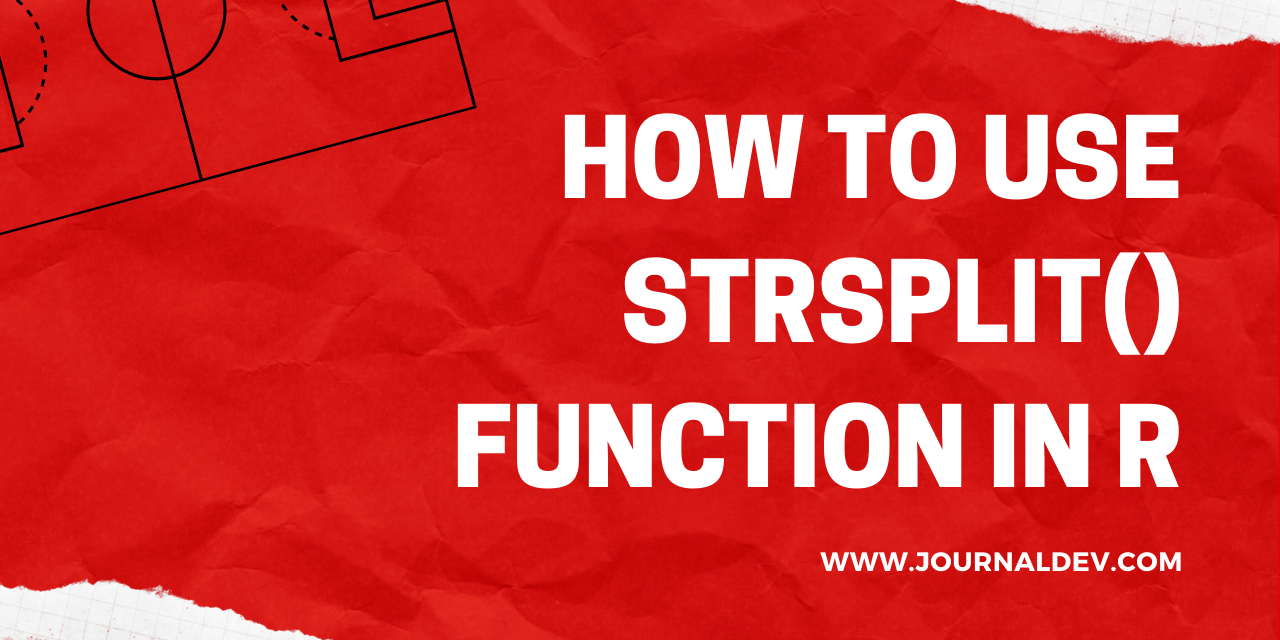# How to use strsplit() function in R?

Published on August 3, 2022By Prajwal CN
Developer and author at DigitalOcean.While we believe that this content benefits our community, we have not yet thoroughly reviewed it. If you have any suggestions for improvements, please let us know by clicking the “report an issue“ button at the bottom of the tutorial.

As a programmer, you may need to work on tons of strings. You will perform concatenation and splitting of them very often. There comes the strsplit() function in R. In a previous article, we have discussed the paste() function to concatenate the strings. Now, let’s see how we can split a string vector using the strsplit().

strsplit() is an exceptional R function, which splits the input string vector into sub-strings. Let’s see how this function works and what are all the ways to perform splitting of the strings in R using the strsplit().

## Strsplit() Function Syntax

Strsplit(): An R Language function which is used to split the strings into substrings with split arguments.

``````strsplit(x,split,fixed=T)
``````

Where:

• X = input data file, vector or a stings.
• Split = Splits the strings into required formats.
• Fixed = Matches the split or uses the regular expression.

## Use strsplit() function in R - Implementation

In this section, let’s see a simple example that shows the use case of the strsplit() function. In this case, the strsplit() function will split the given input into a list of strings or values.

Let’s see how it works.

``````df<-("R is the statistical analysis language")
strsplit(df, split = " ")
``````

Output =

``````"R" "is" "the" "statistical" "analysis" "language"
``````

We have done it! In this way, we can easily split the strings present in the data. One of the best use cases of strsplit() function is in plotting the word clouds. In that, we need tons of word strings to plot the most popular or repeated word. So, in order to get the strings from the data we use this function which returns the list of strings.

### 1. Using strsplit() function with delimiter

A delimiter in general is a simple symbol, character, or value that separates the words or text in the data. In this section, we will be looking into the use of various symbols as delimiters.

``````df<-"get%better%every%day"
strsplit(df,split = '%')
``````

Output =

``````"get" "better" "every"  "day"
``````

In this case, the input text has the % as a delimiter. Now, our concern is to remove the delimiter and get the text as a list of strings. The strsplit() function has done the same here. It removed the delimiter and returned the strings as a list.

### 2. strsplit() function with Regular Expression delimiter

In this section, we will be looking into the splitting of text using regular expressions. Sounds interesting? Let’s do it.

``````df<-"all16i5need6is4a9long8vacation"
strsplit(df,split = "[0-9]+")
``````

Output =

``````"all" "i" "need" "is" "a" "long" "vacation"
``````

In this example, our input has the numbers lies between 0-9. hence we used the regular expression as [0-9]+ to split the data by removing the numbers. The strsplit() function will return a list of strings as output as shown above.

### 3. Split each character in the input string

Till now, we have came across various types of splitting a given string. Now, what if we want to split each and every character of the string? Well, we use the strsplit() function with different split argument to extract each character.

Let’s see how it wokrs.

``````df<-"You can type q() in Rstudio to quit R"
strsplit(df,split="")
``````

Output =

``````"Y" "o" "u" " " "c" "a" "n" " " "t" "y" "p" "e" " " "q" "(" ")" " " "i"
"n" " " "R" "s" "t" "u" "d" "i" "o" " " "t" "o" " " "q" "u" "i" "t" " "
"R"
``````

### 4. Splitting the dates using strsplit() function in R

The another best application of the strsplit() function is, splitting the dates. This use case is so cool and worth doing it. In this section, let’s see how this works.

``````test_dates<-c("24-07-2020","25-07-2020","26-07-2020","27-07-2020","28-07-2020")
test_mat<-strsplit(test_dates,split = "-")
test_mat
``````

Output =

`````` "24"   "07"   "2020"

"25"   "07"   "2020"

"26"   "07"   "2020"

"27"   "07"   "2020"

"28"   "07"   "2020"
``````

You can see a good looking output right? Using this function, we can create numerous splits from the input strings or data as well. You can also convert the dates into matrix format.

``````matrix(unlist(test_mat),ncol=3,byrow=T)
``````

Output =

``````     [,1]  [,2]  [,3]
[1,] "24" "07" "2020"
[2,] "25" "07" "2020"
[3,] "26" "07" "2020"
[4,] "27" "07" "2020"
[5,] "28" "07" "2020"
``````

You can see the above results where we have created a matrix from the split data. Ba cause organising the data is very important for further process. Merely splitting the text doesn’t make any sense unless it is transformed or organised to a reliable form like above sample.

## Conclusion

Well, we are at the end of the article and I hope you now have a better understanding about the working and use cases of the strsplit() function in R. This function is widely used and most popular in terms of splitting the strings. That’s all for now. Will be back with another function another day.

More study: R documentationDeveloper and author at DigitalOcean.